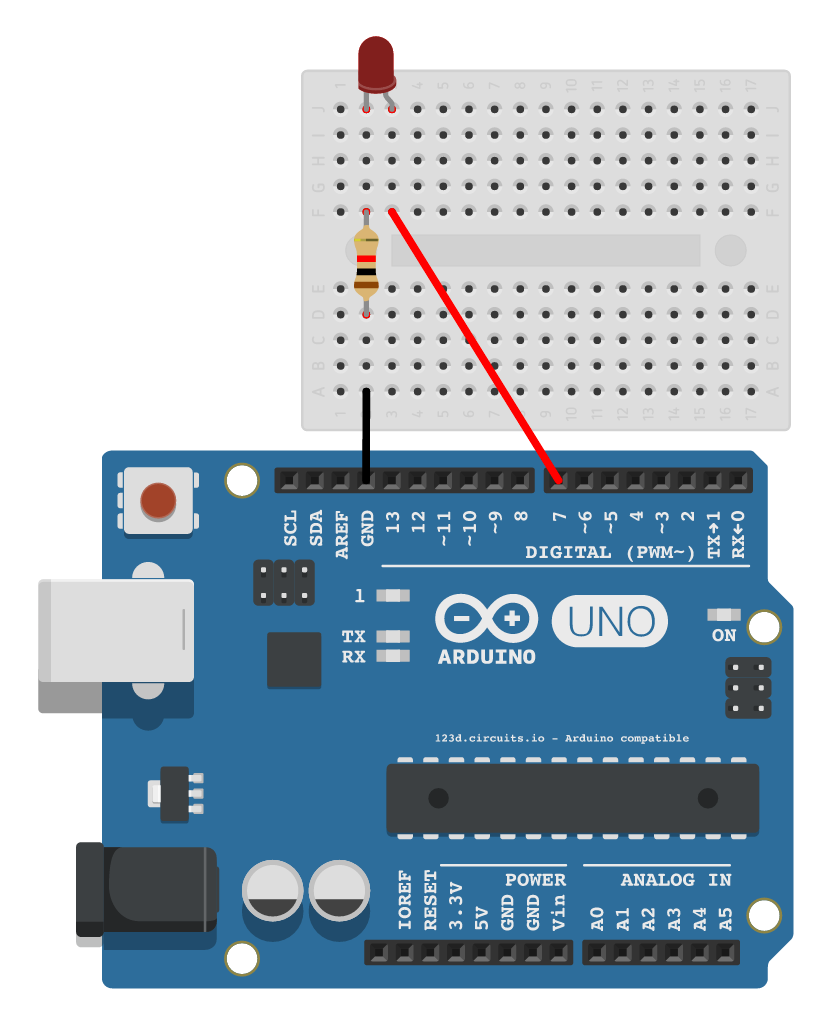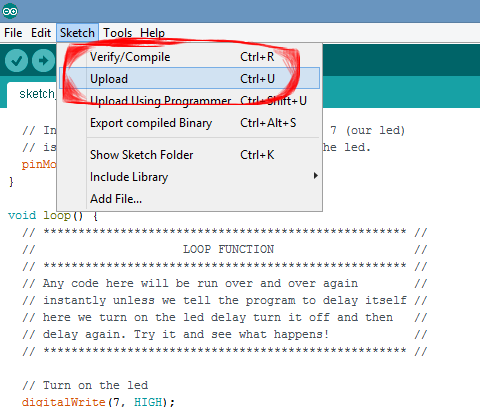arduino‎ > ‎

### lesson1flashingled

Flashing Lights

Overview

In this tutorial you'll create a simple circuit that can turn a light on and off. Below you'll find a diagram and the code.

Set up

2. Use a USB wire to connect your Arduino board to your computer

3. Select the Arduino under Tools > Port

Schematic

When you setup the Arduino it's very important that all the wires go into the same spots as shown below. Also LEDs can only work on one direction. The long side should be connected to pin 7.

Things connect on the breadboard by sharing the same column. In the below schematic one side of the LED is connected to pin 7 and the other is connected to GND (ground by way of a resistor).Code

In this project we're going to learn two new commands.

1. pinMode

The pinMode command tells the Arduino if a in is input or output. To use it we tell it first a pin on the Arduino and second INPUT or OUTPUT. Here is an example...

// here we set pin 7 to OUTPUT
pinMode(7, OUTPUT);

// Here we set pin 4 to INPUT
pinMode(4, INPUT);

1. digitalWrite

The digitalWrite command either sends or stops sending electricity to a pin. To use it we tell it first a pin on the Arduino and second HIGH or LOW where HIGH means ON and LOW means OFF. Always remember that we cannot write to a pin that isn't set to output.

// Here we turn pin 7 on.
digitalWrite(7, HIGH);

// Here we turn pin 4 off.
digitalWrite(4, LOW);

With these two commands use the code below to program your arduino! Simply copy this file into your Arduino editor and upload it!

COPY THIS CODE BELOW
 void setup() { pinMode(7, OUTPUT); } void loop() { digitalWrite(7, HIGH); delay(1000); digitalWrite(7, LOW); delay(1000); }Stretch Goals

1. Can you get the LED to flash twice as fast?

2. Can you get the LED to flash for one second every ten seconds?